# Pointwise product of functions

## Definition

### For two functions

Suppose$f$ and$g$ are functions. The pointwise product (often simply called the product) of the functions, denoted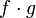$f \cdot g$, or sometimes simply as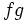$fg$, is defined as the function:$\! x \mapsto f(x)g(x)$

In other words, every element is sent to the product (in the sense of multiplication) of the values of$f$ and$g$ at that element.

The domain of the pointwise product of$f$ and$g$ is defined as the intersection of the domain of$f$ and the domain of$g$. This is necessary, because, for a pointwise product to make sense, both functions must be defined at the point.

### For multiple functions

Suppose$f_1, f_2, \dots, f_n$ are functions. The pointwise product of these, denoted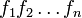$f_1f_2 \dots f_n$ or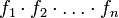$f_1 \cdot f_2 \cdot \dots \cdot f_n$, is defined as the function: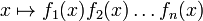$\! x \mapsto f_1(x)f_2(x) \dots f_n(x)$

In other words, every element is sent to the product (in the sense of multiplication) of the values of$f_1, f_2, \dots, f_n$ at that element.

The domain of the pointwise product of a bunch of functions is defined as the intersection of the domains of all the functions.

## Relation with various operations

### For two functions

Below we discuss how to perform various operations on the pointwise product$f \cdot g$ of$f$ and$g$, given knowledge of how to perform the operations on$f$ and$g$ individually.

Operation Verbal description How it's done
Graph$f \cdot g$ We are given the graphs of f and g (without necessarily having algebraic, numerical, or verbal descriptions of the functions) and we need a geometric method to sketch the graph of$f \cdot g$ too tricky?
Obtain explicit expression for$f \cdot g$ We are given algebraic expressions for$f$ and$g$ and need an explicit algebraic expression for$f \cdot g$. Part of a general procedure: finding pointwise combinations of functions by plugging in expressions. See also the piecewise case: finding pointwise combinations of piecewise functions by plugging in expressions.
Find limit of$f \cdot g$ at a point We know how to find limits of$f,g$ at points. limit of products is product of limits
Differentiate$f \cdot g$. We know how to differentiate$f$ and$g$ individually, we need to differentiate$f \cdot g$. product rule for differentiation:$(f \cdot g)' = (f' \cdot g) + (f \cdot g')$.
Integrate$f \cdot g$. We want to integrate$f \cdot g$ in terms of integration of simpler functions. We can try integration by u-substitution or integration by parts.